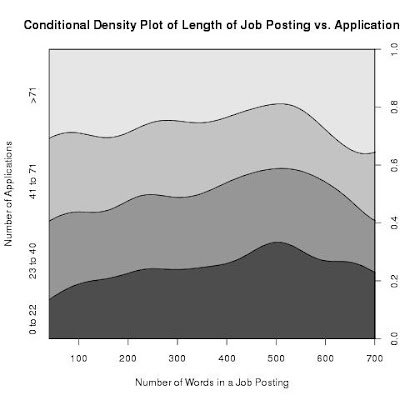## Saturday, October 16, 2010

### A better visualization of job posting length vs application

Here's a plot that should have been included in part three of Mining Jobmine. It describes the change in distribution of applications as the length of job posting changes. For each x-value representing a certain length of job posting, the plot gives an estimate on the portion of jobs that has 0 to 22 applications, 23 to 40 applications, 41 to 70 applications and >71 applications. The end points are chosen to be the 25th, 50th and 75th percentiles of the number of applications.The size of the "0 to 22 applications" category increases steadily as the length of job posting increases from 30-ish to around 500, indicating a drop in application. But as the length of job posting increases beyond 500 words, the size of the bottom-most category decreases. This decrease is offset by an increase in the size of the ">71" category. My guess is that jobs with really long job postings are ones where multiple positions are advertised (e.g. Google job posting...).

Compared to what I had before, this is a much better way of visualizing the correlation between length of job posting and application.

End of Entry

### Trains and German Tanks: a Probability Problem

This problem had bugged me for quite a while, and since many people had contributed to solving it, I thought I should write it up. It's a problem that first came up in an introductory probability course, and was used to teach us the concept of maximum likelihood. Try it yourself before reading the solutions if you like probability puzzles...

Problem Statement

You're standing outside by a railroad. A train pass by, and you realize that it is train #m. You know that the company that operates the trains (Viarail, CN, or whatever) numbers their trains sequentially, so that there is a train #1, #2, #3, all the way up to train #n. Assume that each of the n trains is equally likely to pass by where you stood. Now, given m, the train number that you just saw, estimate n, the total number of trains owned by the company.

You don't get much information from this problem: the only thing you get is the value of m, and you have to guess n. The ambiguity comes from not knowing how our guesses will be judged: should we maximize the probability of guessing n correctly? Should we minimize squared error? Or perhaps we should minimize absolute variance. Each of these different ways of measuring how good our guess is will lead to a different solution.

Solution #1: Maximizing the Probability of Being Right

This was the way my professor interpreted the problem. To maximize our chances of guessing n correctly, we would use maximum likelihood. We choose a guess for n so that P(M=m|N=n) is maximal. Note n has to be at least as big as m, and P(M=m|N=n)=1/n for n>=m, which gets smaller as n increases. So we would pick the value m to be our estimate for n.

This is an unintuitive answer. Again, it's because this solution is only optimal if you only care about guessing correctly, with no credit given for getting close to the real value of n. Run a simulation, and you will see that guessing n=m will indeed maximize your chances of being correct.

Solution #2: Minimizing Squared Error

After some discussions with Greg, I did a simulation were n is drawn from a uniform distribution from 1 to something large, then m was drawn from a uniform distribution from 1 to n. Then we estimated n using km for various values of k, to see what values of k gave the most number of correct guesses (n=km), and also the least square error. As mentioned, setting k=1 (our maximum likelihood estimate) gave the most number of correct guesses, but a different value of k gave the best least square error: k=1.5.

This is a strange value, and it confused me for the longest time. Fortunately, William was able to derive this value using math. We want to minimize E((n-kM)^2) where M~U(1,n), noting that E(M)=(n+1)/2 and E(M^2) = Var(M) + E(M)^2. Expanding E((n-kM)^2), setting its derivative with respect to k to zero, then solving for k gives k = (3n^2+3n)/(2n^2+3n+1), which is approximately 1.5 for large enough n.

Solution #3: Minimizing Absolute Variance

This is relatively simple: set E(n-kM)=0 to get that k should be approximately 2. Amusingly enough, Paul and Kevin pointed out that a generalization of this problem actually came up in real life. Apparently Germans in WWII numbered their tanks sequentially, and so the Western allies were able to use statistical techniques to estimate the number of tanks they had. See http://en.wikipedia.org/wiki/German_tank_problem if you're interested.

Conclusions

The optimal estimate of a variable depends on how you penalize errors (i.e. what statisticians call the loss function). Numbering things sequentially can be really, really dumb. Having nerdy friends who can solve your problems is awesome.

End of Entry